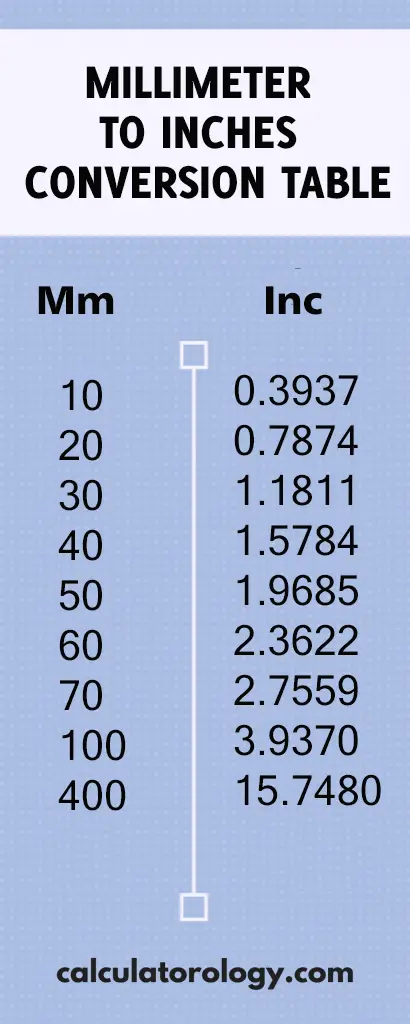## 1.1811023622 inches

### 30 mm to inches

This conversion calculator is used to convert the value in millimeters (mm) to the values in inches (in). To convert 30 mm to inches, it is important to determine the number of inches equivalent to 30 mm. When using the converter, simply enter the value 30 (mm) in the blank text field and then press the ‘Convert’ button. This prompts the calculator to give quick and accurate results based on what is entered in the text field.

#### How many inches in 1 millimeter?

This converter uses a simple formula to convert the values in millimeters to inches.

1 millimeter = 0.03937007874 Inch

This means that there are 0.03937007874 inches in one millimeter. It can also be converted into decimals and shown as;

1 mm = (1/25.4) = 0.03937007874 inches.

D (“) = D (mm)/25.4

Therefore, to determine the distance in inches, you will divide the distance in millimeters by 25.4.## 30mm to Inches Conversion

 mm inches

#### How to convert 30mm to inches

Since 1 millimeter is equivalent to 0.03937007874 inches, how many inches are there in 30 millimeters?

If 1 mm = 0.03937007874 inches,

30mm =? Inches

= (30 x 0.03937007874) inches

= 1.1811023622inches.

This implies that there are 1.1811023622inches in 30 millimeters.

.You can still use the conversion calculator to perform more calculations using different values. The ‘Reset’ button is used to erase all information of the previous calculations to create a new platform for other calculations.

30 mm to inches can also be determined using the Millimeter to Inches conversion table. It gives the conversion results of millimeters to inches based on a range of 0.01mm to 100mm.

##### Definitions

Millimeter is a length unit in metric measurements, abbreviated as “mm”. It is often used in measuring small distances in the fields of machining or engineering.

Inches

It is a US customary and imperial measurement system that is used widely and is abbreviated as (in) or using the symbol (“) - double prime.